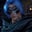Related Tags

python
communitycreator

# How to find the largest element in an array in PythonGutha Vamsi Krishna

Given an array with numerical values, the largest element can be found in multiple ways.

### Example

Input: [20, 49, 1, 99]

Output: 99

### Solution

We can use solve this problem in the following ways in Python:

1. Using a for-in loop
2. Using max()
3. Using sort()

### 1. Using a for-in loop

• We will use a for-in loop and a variable that stores the largest element to solve this.
• Initialize a list lst with [20, 49, 1, 99].
• Initialize the largest variable with the first element in the list.
• Use the for-in loop to traverse every element in the list, and check if the current element i is greater than the value present in the largest variable.
• If it is, then assign the value in i to the variable largest.
• After the loop ends, largest will store the largest element in the list.
#initialize list
lst = [20, 49, 1, 99]

#initialize largest with first element
largest = lst

#traverse the array
for i in lst:
if i>largest:
largest = i

print(largest)

### 2. Using max()

We will use Python’s built-in function max() to solve this problem.

• We will pass the list lst as a parameter to the function max(), which returns the largest element in the list.
#initialize list
lst = [20, 49, 1, 99]

#returns largest element
largest = max(lst)

#print largest in the list
print(largest)

### 3. Using sort()

We will use the sort() method to solve this.

• Initialize the list lst with [20, 99, 1, 49].
• Sort the list lst with lst.sort(), which sorts the original list in ascending order.
• Since it is in ascending order, the last element lst[-1] in the list is the largest. Print it.
#initialize list
lst = [20, 99, 1, 49]

#sort the list
lst.sort()

#print last element which is largest
print(lst[-1])

RELATED TAGS

python
communitycreator

CONTRIBUTORGutha Vamsi Krishna
RELATED COURSES

View all Courses

Keep Exploring

Learn in-demand tech skills in half the time